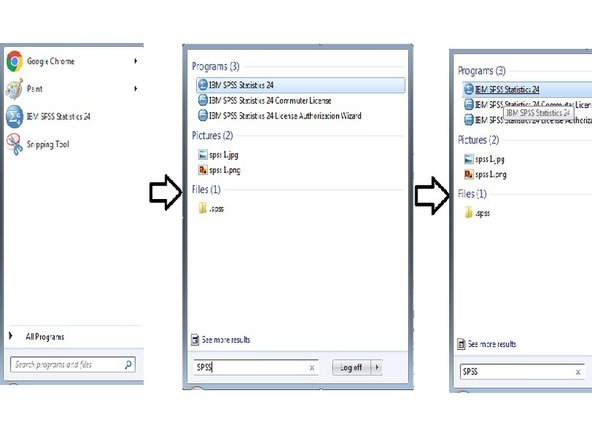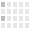Zum Hauptinhalt wechseln

## Einleitung

These instructions show how to use the computer program Statistical Package for the Social Sciences (SPSS), a software system used in analysis of data, for analyzing statistics in Marketing and other disciplines. It explains how to preform a sample t-test, when given a set of data, and explains how to evaluate if the data is significant. These instructions are intended for individuals with a basic background in statistical analysis and research methods.

These instructions were created for use with a Windows computer, and using the SPSS 24 program.

1. ## Schritt 1 Understanding a T-test

• Perform a Sample T-test on SPSS

• Sample T-test: Examines the evidence that the dependent variable is influenced in a meaningful way by the independent variable; if it is, then it is statistically significant.

• Variable: An element, feature, or factor that is liable to vary or change.

• Independent Variable: This variable varies from each statistical test and influences the dependent variable.

• Dependant Variable: The variable that will be measured and affected by the independent variable.

• When to use it: The sample t-test is used to examine whether a sample comes from a particular population when the full population information is not available.

• Sample: Part of a population that is a reasonable size to be analyzed (i.e. a set of 1,000 people given a survey out of a population of 200,000).

• Population: The overall group that a sample is taken from. It usually can’t be represented directly, but instead is represented indirectly through analysis of a sample.

2. ## Schritt 2 Opening SPSS• Click on the start menu of the computer in the bottom left corner of your computer.

• Go to “All Programs.”

• Search “SPSS.”

• Go to “IBM SPSS Statistics."

• Double Click on “IBM SPSS Statistics.”

• The IBM SPSS version represented here is IMB SPSS 24. Different version may be installed on your computer.

3. ## Schritt 3 Inputting Data• Choose between using existing data or entering data manually.

• Existing data may include information already entered into an excel spreadsheet. Data that should be added manually may include data not yet entered into a computer program.

• Go to Step 4: "To use existing data" if you already have the data needed in another program.

• Go to Step 5: "Enter data manually" if your data has not been entered into a program yet.

4. ## Schritt 4 Using existing data• Click on "Open another file" as seen in Figure 1.

• Make sure "Files of Type" is set to "All Files."

• Click "Open" at the bottom of the window.

• Click on the file you want to upload.

• Click "Open" as shown in Figure 2.

• Click "OK" as seen in Figure 3.

• If the data is not in numerical form it will need to be labeled. Go to step 6 to learn for how to do this.

• Skip Step 5 and 6. Go to Step 7.

5. ## Schritt 5 Entering data manually• Click on "New Dataset."

• Click "Open."

• Type in data.

• Data that is not in numerical form will need to be labeled. Go to step 6 to learn for how to do this.

6. ## Schritt 6 Labeling Nominal Data• Switch to "Variable View" as shown in Figure 1.

• Type the label of your variable into the "Label" column as shown in Figure 2.

• Click on the "Value" column shown in Figure 2.

• The following steps are shown in Figure 3.

• Insert the numerical value you would like to use in the "Value" spot.

• Enter the name that the number represents in the "Label" spot.

• Click "Add."

• When complete, click "OK."

7. ## Schritt 7 Understanding Data View• This screen shows all of the data:

• The top row contains the names of each of the variables.

• Each column is a different variable.

• Columns are the vertical boxes.

• Each row is the data connected to an individual.

• Rows are the horizontal boxes.

• Click on “Variable View” at the bottom left of the page shown in Figure 1.

8. ## Schritt 8 Understanding Variable View• In "Variable View":

• The first column, “Name,” states the name of the variable.

• The second column, “Type,” indicates the data type of the variable.

• The third column, “Width,” indicates the number of characters available for the variable values.

• The fourth column, “Decimals,” controls the number of digits after the decimal place.

• The fifth column, “Label,” is used to make any necessary notes.

• The sixth column, “Values,” provides a key for what the numbers of a numeric variable represent.

9. ## Schritt 9 Understanding Variable View Continued• The seventh column, “Missing,” allows a choice of including or excluding values from analysis.

• The eighth column, “Columns,” indicates the number of columns a variable has.

• The ninth column, “Align,” indicates the alignment of the variables.

• The tenth column, “Measure,” indicates the level of measurement of the variable.

• The last column, “Role,” defines the role the variable will play in the analysis.

10. ## Schritt 10 Entering Data and Variable• If you loaded existing data, ignore this step and skip to step 11.

• Make sure you are in “Variable View” by checking the bottom left corner of your screen.

• Put your variable name in the upper left hand cell (no spaces) and make sure the measure is set to “Scale” as seen in Figure 1.

• Switch to “Data View” as seen in Figure 2.

• While in "Data View," plug in the data points under the column you just labeled as seen in Figure 3.

11. ## Schritt 11 Performing a T-test• Click the “Analyze” tab in the upper left hand corner as seen in Figure 1.

• Click on “Compare Means.”

• Click “One Sample t-test.”

• Move the dependent variable over using the arrow in the middle of the screen as seen in Figure 2.

• Fill in the test value spot with the average of the population.

• Click “OK.”

12. ## Schritt 12 Understanding the Results• The first chart is the Descriptive Statistics:

• The first column is the number of participants.

• The second column is the mean.

• Mean: the average of a set of values.

• The third column is the Standard Deviation.

• Standard Deviation: a measure of the expected deviation of the results.

• The fourth column is the Standard Error of the mean.

• Standard Error: the distribution of sample mean around the population mean.

13. ## Schritt 13 Understanding the Results Continued• The second chart is the t-test:

• This chart will be helpful to determine whether the results are statistically significant.

• The first column is the t score of the data.

• The second column is the degrees of freedom.

• The third column is the sig value of the data.

• P-value / sig.: The number that says where on a standard bell curve a value needs to be in order to be statistically significant [ex.: p-value of .05 means that a value must be outside the middle 95% of a bell curve to be statistically significant] (if we use this, we should probably have an image with it)

• The final columns are the ranges inside the confidence intervals.

• Confidence Interval Range: an interval that consist of a range of values that are likely to have the unknown parameter.

14. ## Schritt 14 Analyzing Results

• Determine whether to reject the null hypothesis by looking at the sig value. If the sig value is less than the alpha value, the null hypothesis is rejected. Another way to determine whether to reject the null hypothesis is by looking at the confidence intervals. If 0 is not within these intervals, you can reject the null hypothesis.

• Alpha Value: the probability of rejecting the null hypothesis given that it is true; often equal to the p-value

• Null Hypothesis: the idea that there is no significant difference between specified populations, any observed difference being due to sampling or experimental error

• Alternative Hypothesis: the idea that there is a significant difference between specified populations, any observed difference being due to a changed variable

15. ## Schritt 15 Interpreting Results

• To interpret the results, look at the p-value and the alpha value. If the p-value is less than the alpha value, the null hypothesis is rejected. If the p-value is greater than the alpha, the null hypothesis is accepted.

• Write your results out on a research document like this: "It is not significant. M = 48.70, SD = 27.01461, t(9) = 5.701, p < .00, alpha = .05."

• M: mean

• SD: standard deviation

• t(9): "t" means t-test; "(9)" is the degrees of freedom, which is 9 in this case

• p: p-value

• alpha: alpha value

• Write this for each of the independent and dependent variables.

Ziellinie

3 weitere Nutzer haben diese Anleitung absolviert.

### Autor

#### mikaylalynnnelson

Mitglied seit: 25.03.2017

285 Reputation

1 Anleitung geschrieben

### Team

#### Hot Messes Mitglied von Hot Messes

Community

4 Mitglieder

1 Anleitung geschrieben

### 0 Kommentare

#### Kommentar hinzufügen

Statistik anzeigen:

Letzten 24 Stunden: 0

Letzten 7 Tage: 0

Letzten 30 Tage: 1

Insgesamt: 2,029